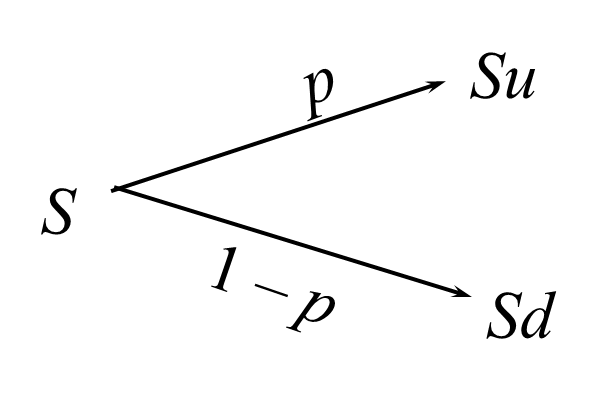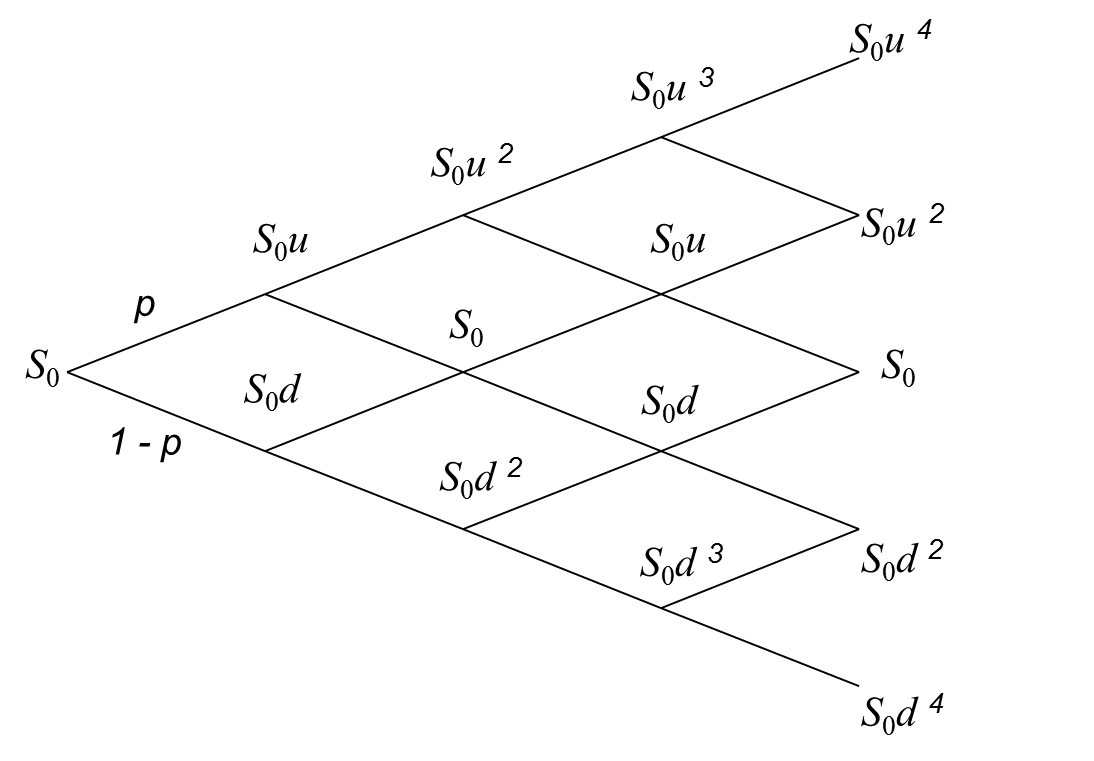# Constructing Binomial Tree to Describe Stock Price Movement

The future price movement of a stock can be approximated using a binomial tree. In a binomial tree, a stock can

Let’s say the current stock price is S. The price of the stock can either move or move down. We will refer to the up movement as u and a down movement as d. A down movement d will then be given by d where d is assumed to be equal to 1/u. We also need the probability of an up move (p) and the probability of a down move (1 - p).

The following diagram shows the price movement in a small time period Δt.From each node at Su and Sd, the prices can again take two possible paths.

• Su can move to Suu or Sud. Note that Sud will be the same as S (S*u*1/u).
• Sd can move to Sdu (or just S) or Sdd.

This way a binomial tree can be built for multiple periods. The following diagram shows the binomial tree for 4 periods.Let’s take an example to understand these values.

Initial stock price, S = $20 u = 1.02 d = 1/1.02 Probability of up move, p = 0.60 Probability of down move, (1-p) = 0.40 The possible stock values for period 1: Su = 20*1.02 = 20.40 with a probability of 0.60 Sd = 19.60 with a probability of 0.40 The possible stock values for period 2: Suu = 20.81 with a probability of 0.60*0.60 = 0.36 S =$20 with a probability of 2*0.60*0.40 = 0.48

Sdd = 19.22 with a probability 0.40*0.40 = 0.16

Similarly we can calculate the values for period 3 and period 4. Binomial trees have their application in pricing options and other financial concepts.

The following diagram shows this binomial tree upto two periods.Get smart about tech at work.

As a non-technical professional, learn how software works with simple explanations of tech concepts. Learn more...

# Data Science for Finance Bundle: 43% OFF

Get our Data Science for Finance Bundle for just $29$51.